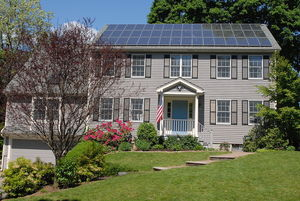Solar Powered Home

Solar Power OverviewFig. 1: Photovoltaic Solar panels on a home. (Source: Wikimedia Commons)

The sun provides a massive amount of energy. This energy can be used as an alternative energy source. This paper focuses on the applications of solar energy to the home.

Is it Feasible?

Although the sun provides orders of magnitude more energy than energy needed for humans, only a percentage can be converted into usable energy. The United Nations Development Programme estimated in 2000 that solar energy could potentially provide per year between 1.575 × 1021 joules and 4.984 × 1022 joules which is 4 to 100 times the present total world energy consumption. [1,2] However, certain factors limit the energy one can practically obtain from the sun. These factors include time variation (which involves seasonal and daily variations), geographic variation (where latitudes closer to the equator receive more solar radiation), weather conditions (specifically the clearness of the sky), and siting options (availability of land for solar energy structures).

So, what about solar energy on a smaller scale? Is it feasible and cost effective for an individual or a family to power a home entirely on solar energy?

I can provide an estimate for the amount of energy required in an average household and an estimate for the amount of energy that can be produced by solar energy and compare the two figures. I am estimating the average household energy consumption by multiplying the average household size in the U.S. (2.62 people in 2002) by the average per capita energy use in the world (3.76812 × 1020 Joules divided by 7 billion people to get 53 billion Joules per person) to get around 140 billion Joules per year. [1,3] I can provide an estimate for the amount of energy that can be produced by solar energy in a household by finding the average square space of rooftops (for solar panels) and multiplying this by the average energy flux received per square space. In 2002, the average square footage of new houses in the United States was 2,340 square feet. Assuming that the average number of floors in a home is 2, we get an estimate of 1,170 square feet of available roof space.  We can multiply this by the average energy flux received by the earth from the sun. The solar constant (1,367 W/m2) is the flux of solar energy on the earth, but to get the average solar flux received by the earth, we project a sphere (the shape of the earth) onto two dimensions which reduces this number by a factor of 4 (surface area of a sphere is 4πr2 to the area of a circle πr2). We get an average flux of 342 W/m2 (= 32 W/ft2) which when multiplied by the available roof space gives us an estimate of 37,440 Watts.  Finally, we multiply this number by a reasonable efficiency of 20% for solar panels to get 7,488 Joules/second. Thus, assuming a generous amount of sunlight per day (10 hours), we get a yearly estimate of 90 billion Joules. This estimate is on the high end and still falls more than two times short of the estimate of average household energy consumption.

Conclusion

So, although solar energy can provide a large portion of the average American's energy needs, another energy source is probably required. However, there are newer solar panels that are more efficient but also more costly, like Tesla's Solar Roof, which would cost \$51,200 for a 70% efficient solar roof or even \$43,700 for a 40% efficient solar roof. 

© Franklin Huang. The author warrants that the work is the author's own and that Stanford University provided no input other than typesetting and referencing guidelines. The author grants permission to copy, distribute and display this work in unaltered form, with attribution to the author, for noncommercial purposes only. All other rights, including commercial rights, are reserved to the author.

References

 "Key World Energy Statistics, 2014," International Energy Agency, 2014, pp. 30-31.

 "Energy and The Challenge of Sustainability," United Nations Development Programme, 2000.

 A. Wilson, and J. Boehland, "Small Is Beautiful: U.S. House Size, Resource Use, and the Environment," J. Ind. Ecol. 9, 277 (2005).

 J. Marshall and R. A Plumb, Atmosphere, Ocean, and Climate Dynamics (Acadmeic Press, 2007), p. 9.

 S. Leasca, "Here's How Much Tesla's Solar Roof Will Really Cost You," Forbes, 16 May 17.